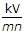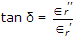# Electronics and Communication Engineering - Materials and Components

Exercise : Materials and Components - Section 5
1.

Assertion (A): Intrinsic resistivity of silicon is lower than that of germanium.

Reason (R): Magnitude of free electron concentration in germanium is more than that of silicon.

Both A and R are true and R is correct explanation of A
Both A and R are true but R is not correct explanation of A
A is true but R is false
A is false but R is true
Explanation:
No answer description is available. Let's discuss.

2.
The units for ∈r areno units
Explanation:

r is only a numeric. Hence no units.

3.
The real part of complex dielectric constant and tanδ for a dielectric are 2.1 and 5 x 10-4 at 100 Hz respectively. The imaginary part of dielectric constant at 100 Hz is
1.05 x 10-3
2.1 x 10-3
5 x 10-3
1.05 x 10-2
Explanation:4.
A copper atom is neutral. Its core has a net charge of
0
+ 1
- 1
+ 2